# ihessian

Hessian of a multivariate, scalar-valued function using the complex-step and central difference approximations.

## Syntax

H = ihessian(f,x0)
H = ihessian(f,x0,hi,hc)


## Description

H = ihessian(f,x0) numerically evaluates the Hessian of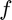with respect to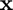at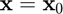using a hybrid of complex-step and central difference approximations with default step sizes of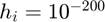(an absolute step size) and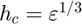(a relative step size), respectively, where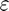is the machine zero.

H = ihessian(f,x0,hi,hc) numerically evaluates the Hessian ofwith respect toatusing a hybrid of complex-step and central difference approximations with user-specified step sizes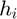and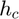, respectively.

## Input/Output Parameters

 Variable Symbol Description Format Input f multivariate, scalar-valued function () 1×1function_handle x0 evaluation point n×1double hi (OPTIONAL) step size for complex-step approximation 1×1double hc (OPTIONAL) relative step size for central difference approximation 1×1double Output H Hessian of with respect to , evaluated at n×ndouble

## Note

• This function requires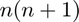evaluations of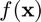.

## Example

Approximate the Hessian of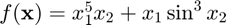at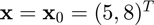using the ihessian function, and compare the result to the true result of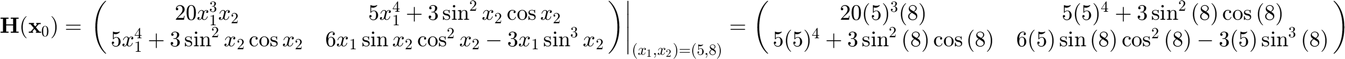Approximating the Hessian,

f = @(x) x(1)^5*x(2)+x(1)*sin(x(2))^3;
x0 = [5;8];
H = ihessian(f,x0)

H =

1.0e+04 *

2.0000    0.3125
0.3125   -0.0014



Calculating the error,

error = H-[20*5^3*8,5*5^4+3*sin(8)^2*cos(8);5*5^4+3*sin(8)^2*cos(8),...
6*5*sin(8)*cos(8)^2-3*5*sin(8)^3]

error =

1.0e-05 *

0.1036    0.0011
0.0011    0.0049